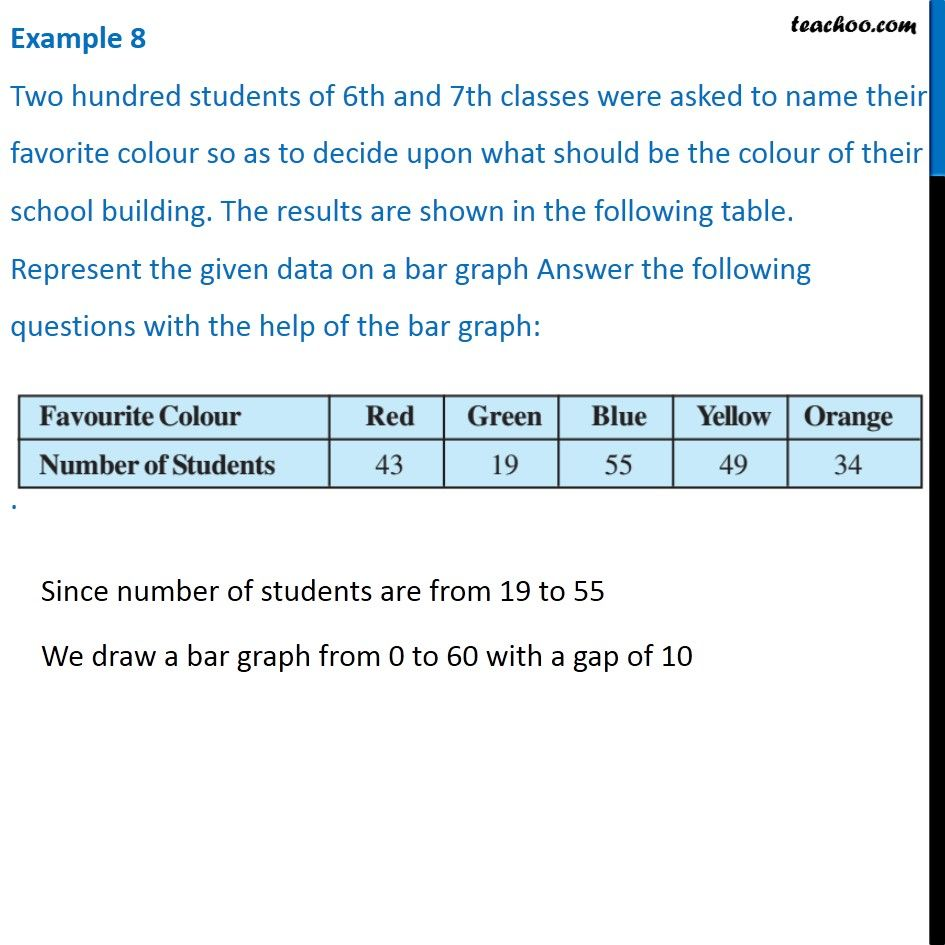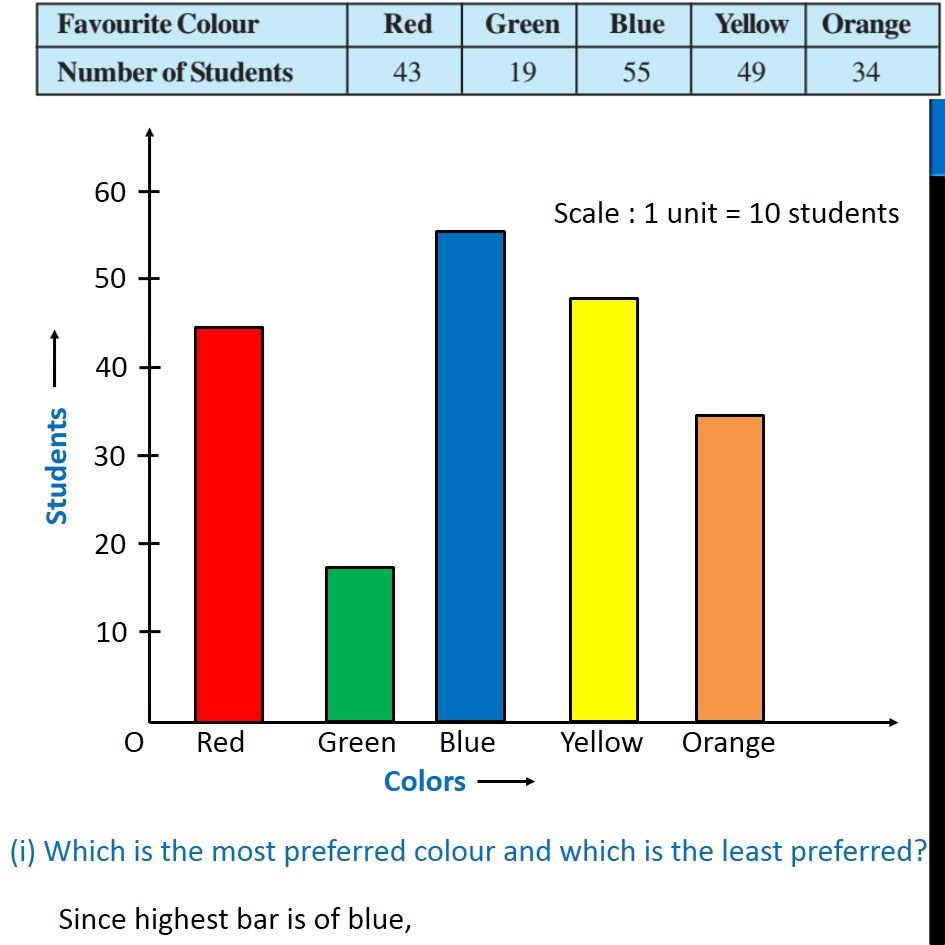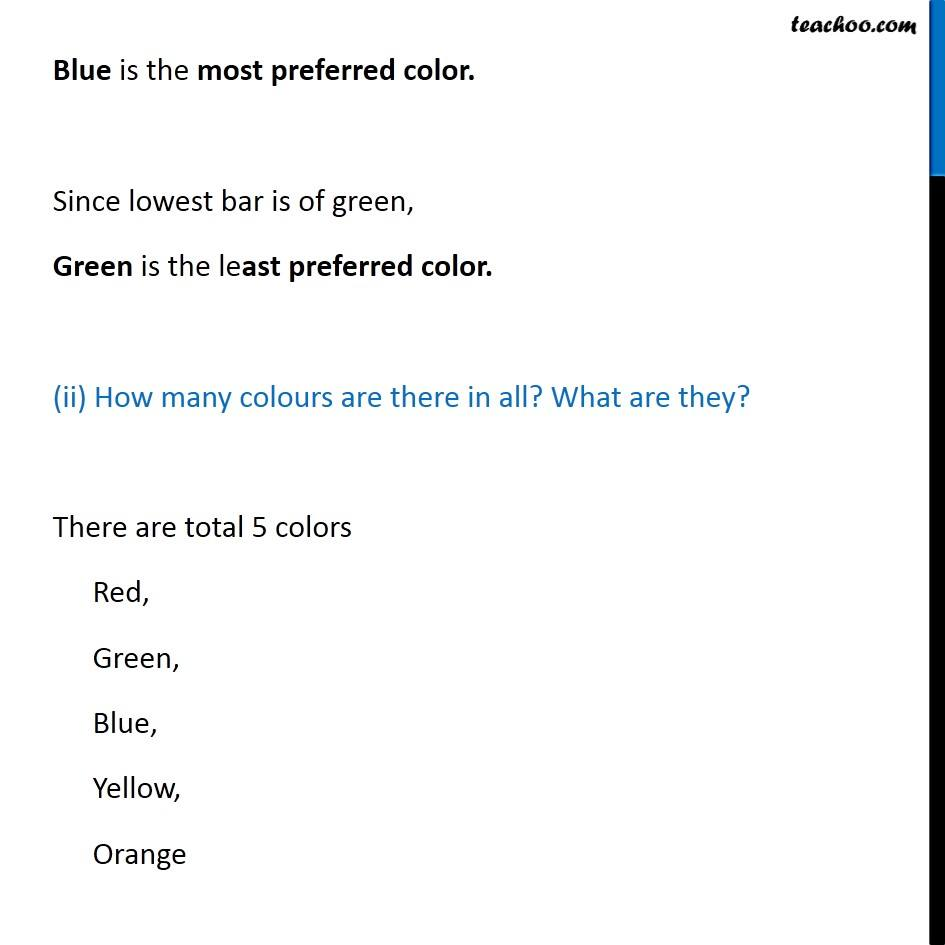Examples

Chapter 3 Class 7 Data Handling
Serial order wiseLearn in your speed, with individual attention - Teachoo Maths 1-on-1 Class

### Transcript

Example 8 Two hundred students of 6th and 7th classes were asked to name their favorite colour so as to decide upon what should be the colour of their school building. The results are shown in the following table. Represent the given data on a bar graph Answer the following questions with the help of the bar graph: Since number of students are from 19 to 55 We draw a bar graph from 0 to 60 with a gap of 10 (i) Which is the most preferred colour and which is the least preferred? Since highest bar is of blue, Blue is the most preferred color. Since lowest bar is of green, Green is the least preferred color. (ii) How many colours are there in all? What are they? There are total 5 colors Red, Green, Blue, Yellow, Orange